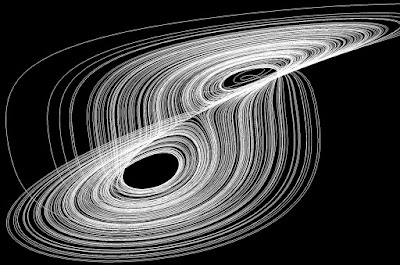# Chaos in Swift with Metal

### Metal is the go-to solution for all things visual in swift. Learn how to use it to impress your friends with cool visualizations. Or at least learn more about metal and swift.

· Mobile Zone · Tutorial
Save
2.02K Views

Inspired by this tweet from Erwin Santacruz, the owner of http://houdinitricks.com, I thought it was high time that I played with some strange attractors in Swift. Strange attractors are equations that represent behavior in a chaotic system and one of the most famous is the Lorenz Attractor which is a simplified model of atmospheric convection.

Although attractors can create some beautiful patterns, the maths behind them is surprisingly simple. For a typical solution for the Lorenz Attractor, given a single point in three-dimensional space, with each time step, its coordinates are updated with the following code:

``````float sigma = 10.0;
float beta = 8.0 / 3.0;
float rho = 28.0;
float deltaX = sigma * (y - x);
float deltaY = x * (rho - z) - y;
float deltaZ = x * y - beta * z;``````

This task is ideally suited to Metal, where individual points can be calculated and rendered super quickly. Although the points are three-dimensional, I decided to stick with a compute shader and render to a two-dimensional texture using my own faux-3D approach. The fundaments of the structure aren't a million miles away from my previous experiments with particle systems under Metal

The project begins with what is effectively an array 222 or 4,194, 304 in size. The first item of this array is given a vector of three random floating point values. Inside the Metal compute shader, an index defines which point, or item in the array, needs to be calculated next using the formula above. The index is incremented with each step.

The faux-3D rendering renders the system rotating around a vertical axis. To do this, the y coordinate of the point maps directly to the screen's y coordinate.  However the screen's x coordinate is the point's x multiplied by the sine of the rotation angle added to the point's z multiplied by the cosine of the rotation angle:

``(thisPoint.x * sin(angle) + thisPoint.z * cos(angle)) * scale``

I've added an implementation of Bresenham's line algorithm to the shader to draw a continual line between the points. Even with the line drawing and millions of points, my iPad Pro runs at 60fps rendering pretty nice results:The final project is available here–enjoy! For some stunningly rendered strange attractors with accompanying maths, check out Strange Attractors on Behance.

Topics:
swift, visualizations, metal

Published at DZone with permission of Simon Gladman, DZone MVB.

Opinions expressed by DZone contributors are their own.